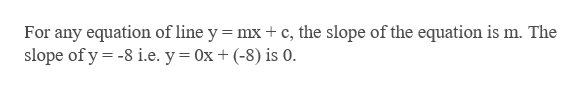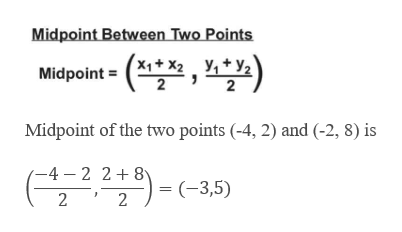# 74.) Find the equation of the line parallel to the line y=-8 and passing through the midpoint of segment joining (-4,2) and (-2,8).78.) show that the x-intercept of the graph of Ax+By=C is (c/a,0)

Question
28 views

74.) Find the equation of the line parallel to the line y=-8 and passing through the midpoint of segment joining (-4,2) and (-2,8).

78.) show that the x-intercept of the graph of Ax+By=C is (c/a,0)

check_circle

Step 1

Given a line y = -8 and a line segment formed by joining points (-4, 2) and (-2, 8).help_outlineImage TranscriptioncloseTo find a line parallel to the given line and passing through the midpoint of the given points fullscreen
Step 2

Note: Two lines are parallel if their slopes are equal. However, they have different y-intercepts.help_outlineImage TranscriptioncloseFor any equation of line y - mx + c, the slope of the equation is m. The slope of y -8 i.e. y = 0x (-8) is 0. fullscreen
Step 3

Further, given that the required line passes thr...help_outlineImage TranscriptioncloseMidpoint Between Two Points y,+y2 X1+x2 Midpoint 2 Midpoint of the two points (-4, 2) and (-2, 8) is -4 - 2 2+8 ' 2 = (-3,5) 2 fullscreen

### Want to see the full answer?

See Solution

#### Want to see this answer and more?

Solutions are written by subject experts who are available 24/7. Questions are typically answered within 1 hour.*

See Solution
*Response times may vary by subject and question.
Tagged in

### Equations and In-equations